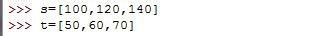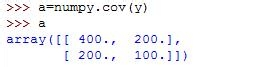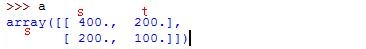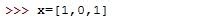• 假如我们现在想要知道身高和体重的关系，我们测量了三个人的身高和体重，身高用s表示，体重用t表示：• 两个向量构成一个向量组：y• 使用numpy.cov方法来求协方差矩阵：• 怎样读懂协方差矩阵呢？400是s和s的协方差（也就是方差）；右上角200是s与t的协方差；100是t与t的协方差（也就是方差）；可见协方差矩阵是一个对称阵• 假如我们再增加一个变量，我们还知道这些人的性别，于是性别就是：• 结果也是这样的：• 这是今天用到的所有代码：

• import numpy

• s=[100,120,140]

• t=[50,60,70]

• y=s+t

• y

• [100, 120, 140, 50, 60, 70]

• y=[s,t]

• y

• [[100, 120, 140], [50, 60, 70]]

• a=numpy.cov(y)

• a

• array([[ 400., 200.],

• [ 200., 100.]])

• x=[1,0,1]

• y=[s,t,x]

• a=numpy.cov(y)

• a

• array([[ 4.00000000e+02, 2.00000000e+02, 0.00000000e+00],

• [ 2.00000000e+02, 1.00000000e+02, 0.00000000e+00],

• [ 0.00000000e+00, 0.00000000e+00, 3.33333333e-01]])